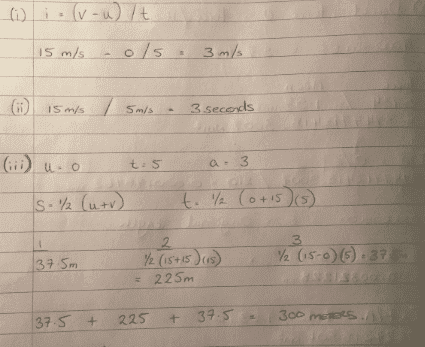# A vehicle of mass 1200 kg accelerates

Member reminded to use the formatting template for all homework help requests

## The Attempt at a Solution

[/B]
Hi All,

I submitted some homework in for marking & received back that on my last question (iii) I had worked out 37.5 wrong, could someone please have a look at my working & advise on where I am making the error.
I'm not sure if the tutor means 37.5 at 1 or 3.
I assume 3 as it says I have questions (i) & (ii) correct.

Kind Regards

A vehicle of mass 1200 kg accelerates uniformly from rest to a velocity of

15 m/s in 5 seconds. It continues at this velocity for 15 seconds before decelerating uniformly at a rate of 5m/s2 to rest. Determine the following:

(i)The acceleration
(ii)The time taken to decelerate from 15 m/s to rest
(iii)The total distance travelled#### Attachments

Last edited by a moderator:

BvU
Homework Helper
Hello NVS,Still having trouble to make decent use of thte template eh ? Look at few other threads.

Your tutor has been very kind. I, for one would have striped (1) too: it's not ##\ \ ## 15 m/s - 0 / 5s ##\ \ ## but ##\ \ ## (15 m/s - 0 m/s) / 5s ##\ \ ## and the result is 3 m/s2 and not 3 m/s

In part (ii) you did OK

Part (iii) must have an error: if at the start you need 5 sec and at the end only 3, the distances covered must be different. Check both !

Is the distance covered 15^2 + 0^2 x 5x2 = 225m?
Or do you need to divide 225 by 2 = 112.5m?

jbriggs444
Homework Helper
Is the distance covered 15^2 + 0^2 x 5x2 = 225m?
Or do you need to divide 225 by 2 = 112.5m?
Show your work. It is no fun looking at a bunch of numbers and multiplication signs and trying to figure out what the student has in mind.

The distance covered can be divided into three sections: Distance traveled while accelerating, distance traveled while moving uniformly and distance traveled while decelerating. Show your calculations and explain your calculations for each of the three phases. Then add them up.

Sorry it was the above question at the top of the page, I have the same question needing help with. But I'll try your method. Thanks.

jbriggs444